# matplotlib.pyplot.axis¶

`matplotlib.pyplot.``axis`(*v, **kwargs)[source]

Convenience method to get or set axis properties.

Calling with no arguments:

```>>> axis()
```

returns the current axes limits `[xmin, xmax, ymin, ymax]`.:

```>>> axis(v)
```

sets the min and max of the x and y axes, with `v = [xmin, xmax, ymin, ymax]`.:

```>>> axis('off')
```

turns off the axis lines and labels.:

```>>> axis('equal')
```

changes limits of x or y axis so that equal increments of x and y have the same length; a circle is circular.:

```>>> axis('scaled')
```

achieves the same result by changing the dimensions of the plot box instead of the axis data limits.:

```>>> axis('tight')
```

changes x and y axis limits such that all data is shown. If all data is already shown, it will move it to the center of the figure without modifying (xmax - xmin) or (ymax - ymin). Note this is slightly different than in MATLAB.:

```>>> axis('image')
```

is ‘scaled’ with the axis limits equal to the data limits.:

```>>> axis('auto')
```

and:

```>>> axis('normal')
```

are deprecated. They restore default behavior; axis limits are automatically scaled to make the data fit comfortably within the plot box.

if `len(*v)==0`, you can pass in xmin, xmax, ymin, ymax as kwargs selectively to alter just those limits without changing the others.

```>>> axis('square')
```

changes the limit ranges (xmax-xmin) and (ymax-ymin) of the x and y axes to be the same, and have the same scaling, resulting in a square plot.

The xmin, xmax, ymin, ymax tuple is returned

`xlim()`, `ylim()`
For setting the x- and y-limits individually.

## Examples using `matplotlib.pyplot.axis`¶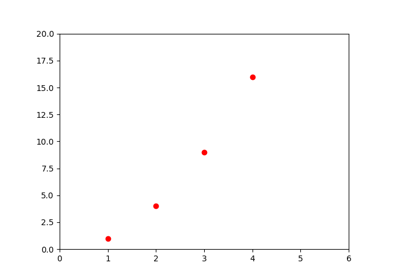Pyplot Formatstr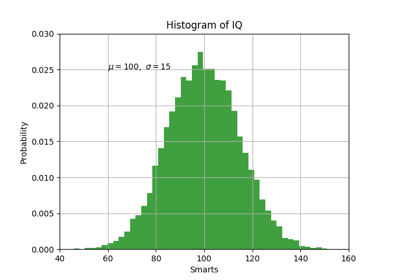Pyplot Text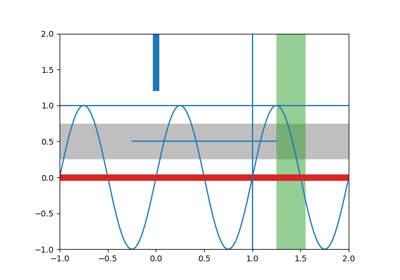axhspan Demo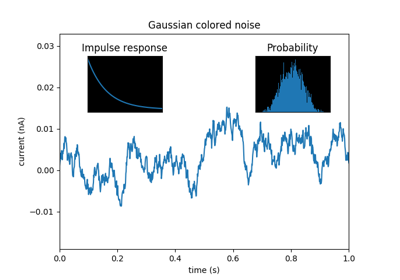Axes Demo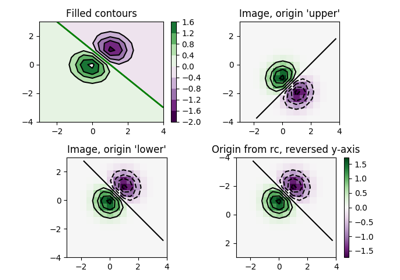Contour Image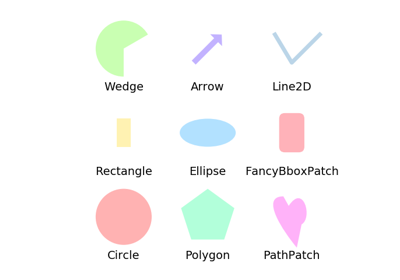Reference for matplotlib artists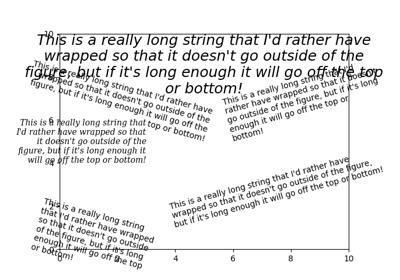Auto-wrapping text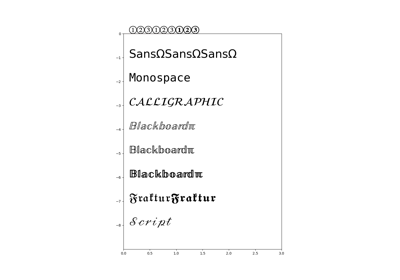STIX Fonts DemoFont Table TTF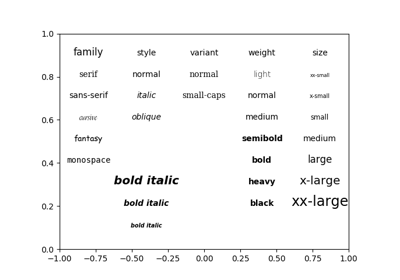Fonts demo (kwargs)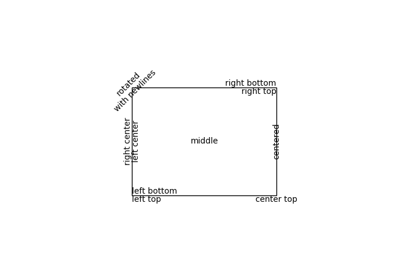Precise text layout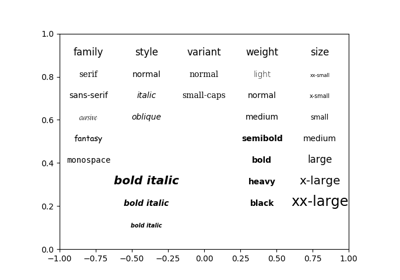Fonts demo (object-oriented style)Interactive functions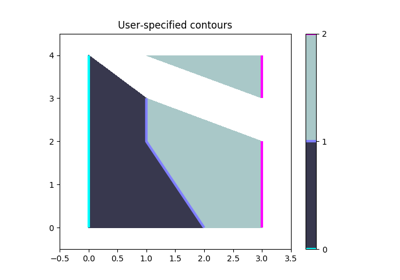Manual ContourCursor Demo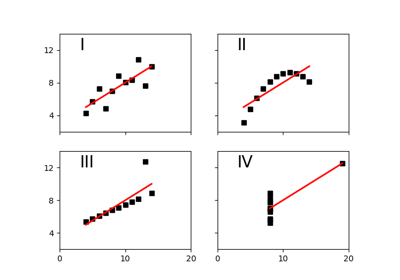Anscombe’s Quartet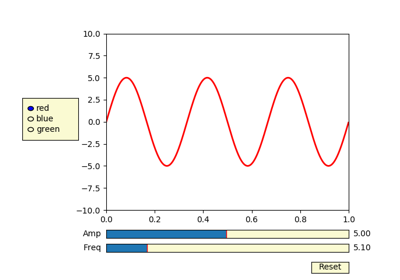Slider Demo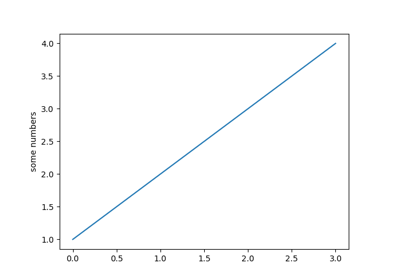Pyplot tutorial